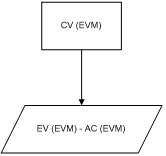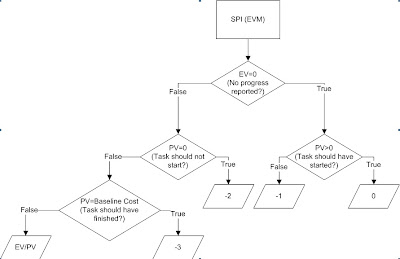# Microsoft Project on Steroids II## 14 Aug Microsoft Project on Steroids II

4. CV (EVM) is EV (EVM) – AC (EVM) (You can use CV if you used BCWP in point 3). For summary tasks, we use the formula (but you could do a rollup-sum):5. SV (EVM) is EV (EVM) – PV (EVM) (You can use SV if you used BCWP in point 3). For summary tasks, we use the formula (but you could do a rollup-sum):6. CPI (EVM):The thresholds used here are only an example. These values can be changed to reflect what you consider good, average, warning, and bad values.

7. SPI (EVM):The thresholds used here are only an example. These values could be changed to reflect what you consider good, average, warning, and bad values.

J